This codelab will show you how to build a reverse image search engine using Milvus and Towhee. The basic idea behind semantic reverse image search is the extract embeddings from images using a deep neural network and compare the embeddings with those stored in Milvus. More details you can refer to the notebook.

Towhee is a machine learning framework that allows for creating data processing pipelines, and it provides predefined operators which implement insert and query operation in Milvus.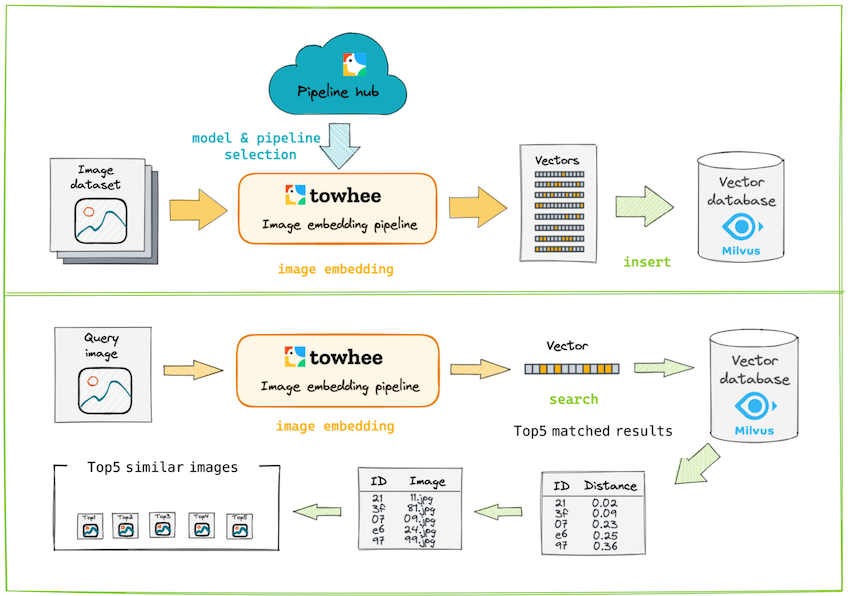First we need to prepare the dependencies and dataset, also the Milvus environment.

## Install Dependencies¶

First we need to install dependencies such as pymilvus, towhee, gradio, opencv-python and pillow.

``````\$ python -m pip install -q pymilvus towhee gradio opencv-python pillow
``````

## Prepare the data

There is a subset of the ImageNet dataset (100 classes, 10 images for each class) is used in this demo, and the dataset is available via Github.

The dataset is organized as follows:

• train: directory of candidate images;
• test: directory of the query images;
• reverse_image_search.csv: a csv file containing an id, path, and label for each image;

``````\$ curl -L https://github.com/towhee-io/examples/releases/download/data/reverse_image_search.zip -O
\$ unzip -q -o reverse_image_search.zip
``````

Let's take a quick look with Python:

``````import pandas as pd

``````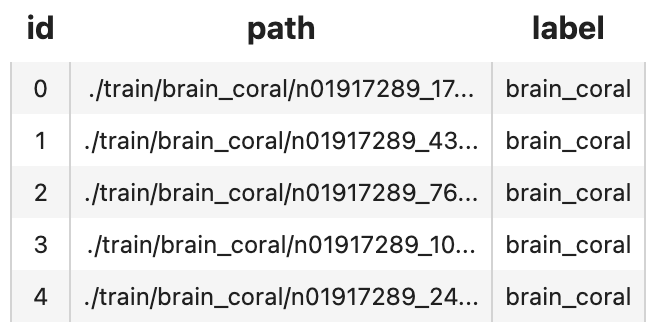To use the dataset for image search, let's first define some helper functions:

• ground_truth(path): ground-truth for each query image, which is used for calculating mHR(mean hit ratio) and mAP(mean average precision);
``````import cv2
import pandas as pd
from towhee._types.image import Image

id_img = df.set_index('id')['path'].to_dict()
label_ids = {}
for label in set(df['label']):
label_ids[label] = list(df[df['label']==label].id)

imgs = []
for re in results:
path = id_img[re.id]
return imgs

def ground_truth(path):
label = path.split('/')[-2]
return label_ids[label]
``````

## Create a Milvus Collection

Before getting started, please make sure you have installed milvus. Let's first create a `reverse_image_search` collection that uses the L2 distance metric and an IVF_FLAT index.

``````from pymilvus import connections, FieldSchema, CollectionSchema, DataType, Collection, utility

connections.connect(host='127.0.0.1', port='19530')

def create_milvus_collection(collection_name, dim):
if utility.has_collection(collection_name):
utility.drop_collection(collection_name)

fields = [
FieldSchema(name='id', dtype=DataType.INT64, descrition='ids', is_primary=True, auto_id=False),
FieldSchema(name='embedding', dtype=DataType.FLOAT_VECTOR, descrition='embedding vectors', dim=dim)
]
schema = CollectionSchema(fields=fields, description='reverse image search')
collection = Collection(name=collection_name, schema=schema)

# create IVF_FLAT index for collection.
index_params = {
'metric_type':'L2',
'index_type':"IVF_FLAT",
'params':{"nlist":2048}
}
collection.create_index(field_name="embedding", index_params=index_params)
return collection
``````

We first extract embeddings from images with `resnet50` model and insert the embeddings into Milvus for indexing. Towhee provides a method-chaining style API so that users can assemble a data processing pipeline with operators.

``````import towhee

collection = create_milvus_collection('reverse_image_search', 2048)
dc = (
.runas_op['id', 'id'](func=lambda x: int(x))
.image_decode['path', 'img']()
.image_embedding.timm['img', 'vec'](model_name='resnet50')
.to_milvus['id', 'vec'](collection=collection, batch=100)
)
print('Total number of inserted data is {}.'.format(collection.num_entities))
``````

### Explanation of Data Processing Pipeline in Towhee

Here is detailed explanation for each line of the code:

• `towhee.read_csv('reverse_image_search.csv')`: read tabular data from csv file (`id`, `path` and `label` columns);
• `.runas_op['id', 'id'](func=lambda x: int(x))`: for each row from the data, convert the data type of the column `id` from `str` to `int`;
• `.image_decode['path', 'img']()`: for each row from the data, read and decode the image at `path` and put the pixel data into column `img`;
• `.image_embedding.timm['img', 'vec'](model_name='resnet50')`: extract embedding feature with `image_embedding.timm`, an operator from the Towhee hub based on pytorch-image-models. This operator supports a variety of image models trained on ImageNet, including `vgg16`, `resnet50`, `vit_base_patch8_224`, `convnext_base`, etc.
• `.to_milvus['id', 'vec'](collection=collection, batch=100)`: insert image embedding features in to Milvus;

Now that embeddings for candidate images have been inserted into Milvus, we can query across it for nearest neighbors. Again, we use Towhee to load the input image, compute an embedding vector, and use the vector as a query for Milvus. Because Milvus only outputs image IDs and distance values, we provide a `read_images` function to get the original image based on IDs and display.

``````(
towhee.glob['path']('./test/w*/*.JPEG')
.image_decode['path', 'img']()
.image_embedding.timm['img', 'vec'](model_name='resnet50')
.milvus_search['vec', 'result'](collection=collection, limit=5)
.select['img', 'result_img']()
.show()
)
``````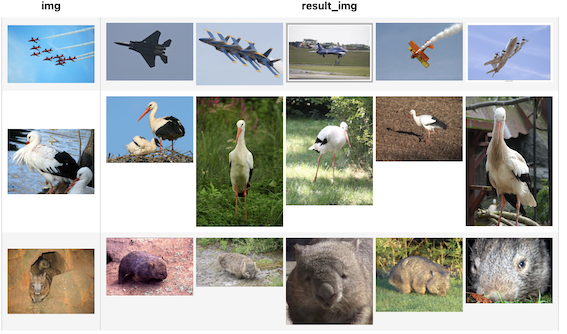We have finished the core functionality of the image search engine. However, we don't know whether it achieves a reasonable performance. We need to evaluate the search engine against the ground truth so that we know if there is any room to improve it.

In this section, we'll evaluate the strength of our image search engine using mHR and mAP:

• mHR (recall@K): This metric describes how many actual relevant results were returned out of all ground-truth relevant results by the search engine. For example, if we have put 100 pictures of cats into the search engine and then query the image search engine with another picture of cats. The total relevant result is 100, and the actual relevant results are the number of cat images in the top 100 results returned by the search engine. If there are 80 images about cats in the search result, the hit ratio is 80/100;
• mAP: Average precision describes whether all of the relevant results are ranked higher than irrelevant results.
``````benchmark = (
towhee.glob['path']('./test/*/*.JPEG')
.image_decode['path', 'img']()
.image_embedding.timm['img', 'vec'](model_name='resnet50')
.milvus_search['vec', 'result'](collection=collection, limit=10)
.runas_op['path', 'ground_truth'](func=ground_truth)
.runas_op['result', 'result'](func=lambda res: [x.id for x in res])
.with_metrics(['mean_hit_ratio', 'mean_average_precision'])
.evaluate['ground_truth', 'result']('resnet50')
.report()
)
``````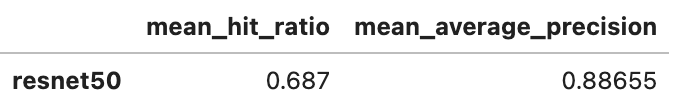The mean HR of all the queries is 0.687 (not a great result). Let's optimize it further.

## Optimization I: embedding vector normalization

A quick optimization is normalizing the embedding features before indexing them in Milvus. This results in cosine similarity, which measures the similarity between two vectors using the angle between them while ignoring the magnitude of the vectors.

``````collection = create_milvus_collection('reverse_image_search_norm', 2048)

dc = (
.runas_op['id', 'id'](func=lambda x: int(x))
.image_decode['path', 'img']()
.image_embedding.timm['img', 'vec'](model_name='resnet50')
.tensor_normalize['vec', 'vec']()
.to_milvus['id', 'vec'](collection=collection, batch=100)
)

benchmark = (
towhee.glob['path']('./test/*/*.JPEG')
.image_decode['path', 'img']()
.image_embedding.timm['img', 'vec'](model_name='resnet50')
.tensor_normalize['vec', 'vec']()
.milvus_search['vec', 'result'](collection=collection, limit=10)
.runas_op['path', 'ground_truth'](func=ground_truth)
.runas_op['result', 'result'](func=lambda res: [x.id for x in res])
.with_metrics(['mean_hit_ratio', 'mean_average_precision'])
.evaluate['ground_truth', 'result']('resnet50')
.report()
)
``````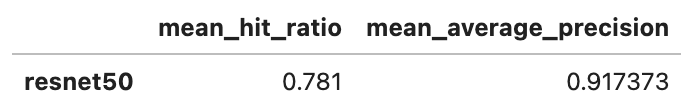By normalizing the embedding features, the mean HR shoots up to 0.781.

## Optimization II: increase model complexity

Another quick optimization is increase model complexity (at the cost of runtime). With Towhee, this is very easy: we simply replace Resnet-50 with EfficientNet-B7, an image classificiation model which has better accuracy on ImageNet. Although Towhee provides a pre-trained EfficientNet-B7 model via `timm`, we'll use `torchvision` to demonstrate how external models and functions can be used within Towhee.

``````import torch
import towhee
from torchvision import models
from torchvision import transforms
from PIL import Image as PILImage

torch_model = models.efficientnet_b7(pretrained=True)
torch_model = torch.nn.Sequential(*(list(torch_model.children())[:-1]))
torch_model.to('cuda' if torch.cuda.is_available() else 'cpu')
torch_model.eval()
preprocess = transforms.Compose([
transforms.Resize(size=224, interpolation=transforms.InterpolationMode.BICUBIC),
transforms.ToTensor(),
transforms.Normalize(
mean=[0.485, 0.456, 0.406],
std=[0.229, 0.224, 0.225]
)
])

def efficientnet_b7(img):
img = cv2.cvtColor(img, cv2.COLOR_BGR2RGB)
img = PILImage.fromarray(img.astype('uint8'), 'RGB')
img = torch.unsqueeze(preprocess(img), 0)
img = img.to('cuda' if torch.cuda.is_available() else 'cpu')
embedding = torch_model(img).detach().cpu().numpy()
return embedding.reshape()
``````

This illustrates how to use a PyTorch model from torch hub. You can follow the previous code when testing your own model against the benchmark.

``````collection = create_milvus_collection('image_search_efficientnet_b7', 2560)

dc = (
.runas_op['id', 'id'](func=lambda x: int(x))
.image_decode['path', 'img']()
.runas_op['img', 'vec'](func=efficientnet_b7)
.tensor_normalize['vec', 'vec']()
.to_milvus['id', 'vec'](collection=collection, batch=100)
)

benchmark = (
towhee.glob['path']('./test/*/*.JPEG')
.image_decode['path', 'img']()
.runas_op['img', 'vec'](func=efficientnet_b7)
.tensor_normalize['vec', 'vec']()
.milvus_search['vec', 'result'](collection=collection, limit=10)
.runas_op['path', 'ground_truth'](func=ground_truth)
.runas_op['result', 'result'](func=lambda res: [x.id for x in res])
.with_metrics(['mean_hit_ratio', 'mean_average_precision'])
.evaluate['ground_truth', 'result']('efficientnet_b7')
.report()
)
``````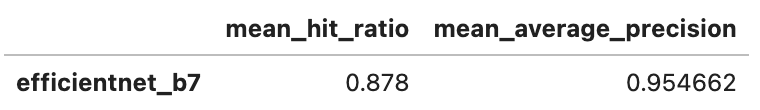By replacing Resnet50 with EfficientNet-B7, the mean HR is raised to 0.878! But the data processing pipeline also gets much slower and takes 28% more time.

We've done an excellent job on the core functionality of our image search engine. Now it's time to build a showcase with interface. Gradio is a great tool for building demos. With Gradio, we simply need to wrap the data processing pipeline via a `search_in_milvus` function:

``````from towhee.types.image_utils import from_pil

with towhee.api() as api:
milvus_search_function = (
api.runas_op(func=lambda img: from_pil(img))
.image_embedding.timm(model_name='resnet50')
.tensor_normalize()
.milvus_search(collection='reverse_image_search_norm', limit=5)
.runas_op(func=lambda res: [id_img[x.id] for x in res])
.as_function()
)